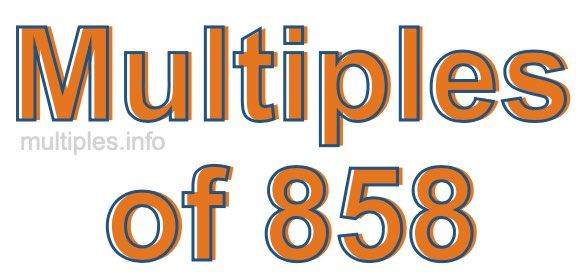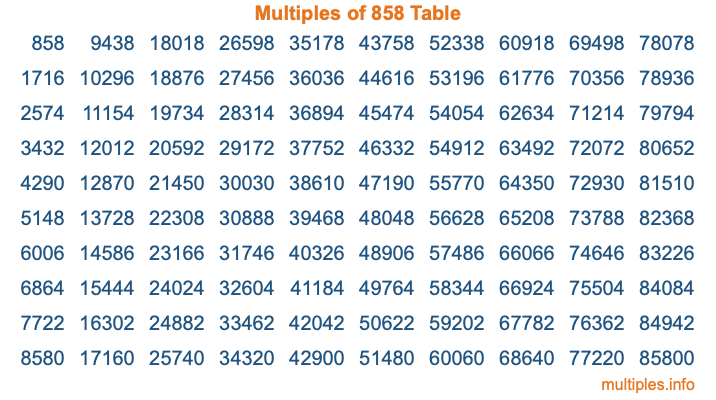Multiples of 858Welcome to the Multiples of 858 page. Here we will first teach you everything you will ever need to know about the multiples of 858, and then give you a study guide summary of everything we taught you to make sure you remember it all. Use this page to look up facts and learn information about the multiples of 858. This page will make you a multiples of eight hundred fifty-eight expert!

Definition of Multiples of 858
Multiples of 858 are all the numbers that when divided by 858 equal an integer. Each of the multiples of 858 are called a multiple. A multiple of 858 is created by multiplying 858 by an integer.

Therefore, to create a list of multiples of 858, you start with 1 multiplied by 858, then 2 multiplied by 858, then 3 multiplied by 858, and so on for as long as you want. Thus, the list of the first five multiples of 858 is 858, 1716, 2574, 3432, and 4290. To see a larger list of multiples of 858, see the printable image of Multiples of 858 further down on this page. We also have a category where you can choose any nth multiple of 858.

Multiples of 858 Checker
The Multiples of 858 Checker below checks to see if any number of your choice is a multiple of 858. In other words, it checks to see if there is any number (integer) that when multiplied by 858 will equal your number. To do that, we divide your number by 858. If the the quotient is an integer, then your number is a multiple of 858.

Is  a multiple of 858?

Least Common Multiple of 858 and ...
A Least Common Multiple (LCM) is the lowest multiple that two or more numbers have in common. This is also called the smallest common multiple or lowest common multiple and is useful to know when you are adding our subtracting fractions. Enter one or more numbers below (858 is already entered) to find the LCM.

Check out our LCM Calculator if you need more details about the Least Common Multiple or if you need the LCM for different numbers for adding and subtraction fractions.

nth Multiple of 858
As we stated above, 858 is the first multiple of 858, 1716 is the second multiple of 858, 2574 is the third multiple of 858, and so on. Enter a number below to find the nth multiple of 858.

th multiple of 858

Multiples of 858 vs Factors of 858
858 is a multiple of 858 and a factor of 858, but that is where the similarities end. All postive multiples of 858 are 858 or greater than 858. All positive factors of 858 are 858 or less than 858.

Below is the beginning list of multiples of 858 and the factors of 858 so you can compare:

Multiples of 858: 858, 1716, 2574, 3432, 4290, etc.

Factors of 858: 1, 2, 3, 6, 11, 13, 22, 26, 33, 39, 66, 78, 143, 286, 429, 858

As you can see, the multiples of 858 are all the numbers that you can divide by 858 to get a whole number. The factors of 858, on the other hand, are all the whole numbers that you can multiply by another whole number to get 858.

It's also interesting to note that if a number (x) is a factor of 858, then 858 will also be a multiple of that number (x).

Multiples of 858 vs Divisors of 858
The divisors of 858 are all the integers that 858 can be divided by evenly. Below is a list of the divisors of 858.

Divisors of 858: 1, 2, 3, 6, 11, 13, 22, 26, 33, 39, 66, 78, 143, 286, 429, 858

The interesting thing to note here is that if you take any multiple of 858 and divide it by a divisor of 858, you will see that the quotient is an integer.

Multiples of 858 Table
Below is an image of the first 100 multiples of 858 in a table. The table is in chronological order, column by column. The first column has the first ten multiples of 858, the second column has the next ten multiples of 858, and so on.The Multiples of 858 Table is also referred to as the 858 Times Table or Times Table of 858. You are welcome to print out our table for your studies.

Negative Multiples of 858
Although not often discussed or needed in math, it is worth mentioning that you can make a list of negative multiples of 858 by multiplying 858 by -1, then by -2, then by -3, and so on, to get the following list of negative multiples of 858:

-858, -1716, -2574, -3432, -4290, etc.

Multiples of 858 Summary
Below is a summary of important Multiples of 858 facts that we have discussed on this page. To retain the knowledge on this page, we recommend that you read through the summary and explain to yourself or a study partner why they hold true.

There are an infinite number of multiples of 858.

A multiple of 858 divided by 858 will equal a whole number.

858 divided by a factor of 858 equals a divisor of 858.

The nth multiple of 858 is n times 858.

The largest factor of 858 is equal to the first positive multiple of 858.

858 is a multiple of every factor of 858.

858 is a multiple of 858.

A multiple of 858 divided by a divisor of 858 equals an integer.

858 divided by a divisor of 858 equals a factor of 858.

Any integer times 858 will equal a multiple of 858.

Multiples of a Number
Here you can get the multiples of another number, all with the same attention to detail as we did for multiples of 858 on this page.

Multiples of
Multiples of 859
Did you find our page about multiples of eight hundred fifty-eight educational? Do you want more knowledge? Check out the multiples of the next number on our list!